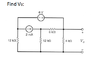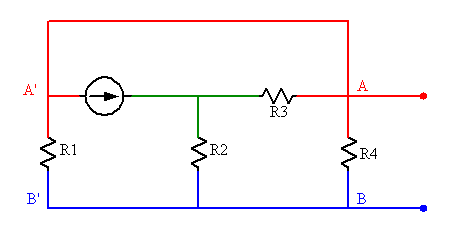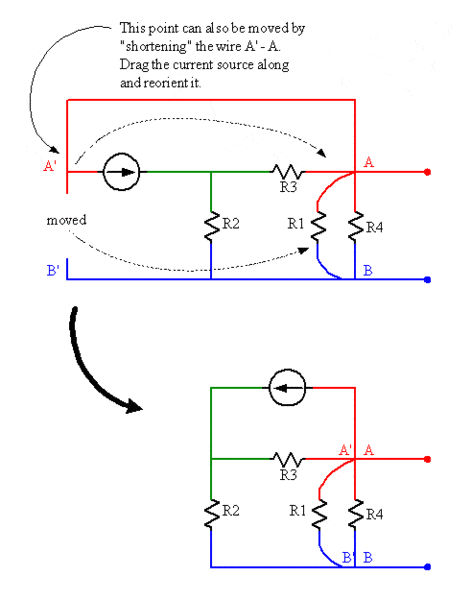# Help finding total resistance in a circuit

• yugeci

## Homework Statement

I attached the question. We have to use superposition to find the value of Vo.

## Homework Equations

Rt = R1 + R2 + R3 + ... + Rn in series.
(1/Rt) = (1/R1 + 1/R2 + 1/R3 + ... + 1/Rn)^-1 in parallel.

Current divider = (Rx/Rt)*Is
Voltage divider = (Vx/Vt)*Vs

## The Attempt at a Solution

To find the value of Vo, I know I have to first replace the voltage source only with a short circuit and find Vo'.

Then remove the current source only with an open circuit and find Vo".

Then Vo = Vo' + Vo".

The problem I have is the setup of the resistors. How do we know what is in parallel/series with what? My teacher solved this question by shifting the resistors around but I did not understand that. Any tips for finding the total resistance where a setup of resistors similar to this would be appreciated.

If I can understand how to find the total resistance I should be able to solve this problem.

#### Attachments

•superpos.png
4.5 KB · Views: 524
I'm getting the wrong answer when I use KCL.

My value of Vo when removing the current source of 2mA is 1.28 V. I believe this is correct.

My value of Vo when removing the voltage source of 6V is 4.36 V. This is most likely incorrect.

If I apply KCL at the node just right of the 2mA source, I treated the 6k and 4k resistors in series, and that combination in parallel with the 12k resistor. With that method the current flowing into the 4k resistor is 1.09 mA. I think that is incorrect.

Did I apply KCL wrongly?

Last edited:
Nodal analysis would be a good approach for this circuit, superposition or not.

If you suppress the current source you're left with one independent node (the voltage source creates a supernode). If you suppress the voltage source there are two independent nodes.

But if you're determined to bang away at it with resistor reductions you'll need to be creative in moving things around to recognize opportunities. Make some drawings, and if you are unsure about whether or not a move is legal, show us your drawing and explain what you want to do.

I'm getting the wrong answer when I use KCL.

My value of Vo when removing the current source of 2mA is 1.28 V. I believe this is correct.
Yup. An exact value can be found: 9/7 V.

My value of Vo when removing the voltage source of 6V is 4.36 V. This is most likely incorrect.

If I apply KCL at the node just right of the 2mA source, I treated the 6k and 4k resistors in series, and that combination in parallel with the 12k resistor. With that method the current flowing into the 4k resistor is 1.09 mA. I think that is incorrect.

Did I apply KCL wrongly?
Yes, yes you didThe suppressed voltage source makes things more complicated. You don't just remove it, you replace it with a short circuit (replace the voltage source with a wire). This changes the topology of the circuit a bit. See if you can tell what happens to that 12k resistor on the left end...

Nodal analysis would be a good approach for this circuit, superposition or not.

If you suppress the current source you're left with one independent node (the voltage source creates a supernode). If you suppress the voltage source there are two independent nodes.

But if you're determined to bang away at it with resistor reductions you'll need to be creative in moving things around to recognize opportunities. Make some drawings, and if you are unsure about whether or not a move is legal, show us your drawing and explain what you want to do.
I know nodal is better. However the teacher wanted us to practice superposition with these examples. I am not very good at rearranging circuits, so I would like to try KCL if possible.

I can't figure out what happens to the 12k resistor... my head is hurting. :p

I know nodal is better. However the teacher wanted us to practice superposition with these examples. I am not very good at rearranging circuits, so I would like to try KCL if possible.
You can still use nodal analysis. Use it twice, once for each source. Obtain the output voltage for each case, sum them later.

I can't figure out what happens to the 12k resistor... my head is hurting. :p
Follow the wires from each end to see what they connect to. You can move a component's connection to any place on a given node. So long as there's a continuous wire leading from the "new" spot to the "old", it's effectively still connected in the same way.

OK so let's suppose the node above the left most 12k resistor is V1. The node just right of the 2mA source is V2, and the one above the 4k resistor is V3. And the ground is a reference node. The left most 12k resistor is connected to V1 and the reference node. So how else can it be "moved"? Likewise how can any other resistor be moved? I think I am misunderstanding something.

I feel embarrassed for not knowing how to solve this equation using nodal analysis as well (I gave that a try). My equations are:

0 = (V3/4k) + (V3-V2)/6k

(V3-V2)/6k = (V2/12k) + (V1/12k)

..and I can't think of a third equation. I'm positive the above 2 equations are incorrect as well. I don't know, I think circuits will layouts of resistors similar to this are tricky to solve.

OK so let's suppose the node above the left most 12k resistor is V1. The node just right of the 2mA source is V2, and the one above the 4k resistor is V3. And the ground is a reference node. The left most 12k resistor is connected to V1 and the reference node. So how else can it be "moved"? Likewise how can any other resistor be moved? I think I am misunderstanding something.

I feel embarrassed for not knowing how to solve this equation using nodal analysis as well (I gave that a try). My equations are:

0 = (V3/4k) + (V3-V2)/6k

(V3-V2)/6k = (V2/12k) + (V1/12k)
Where's the term for the 2mA current source?

..and I can't think of a third equation. I'm positive the above 2 equations are incorrect as well. I don't know, I think circuits will layouts of resistors similar to this are tricky to solve.

When the 6V voltage source is in place your V1 node is not independent. It is directly dependent upon your V3 node as the voltage source fixes V1 = V3 - 6. This is what is known as a Supernode. Both nodes are treated as a single node as far as writing KCL goes, with "V3 - 6" replacing "V1" where you'd normally write "V1" in your equation.

When the 6V source is suppressed (set equal to 0V, not removed from the circuit!), then your V1 = V3; the two nodes again are one node, so your nodal equation for that node must include all the connected branches. Choose one variable to represent the node name. (I think I'd choose "Vo").

If you want to simplify the circuit for the 6V source suppressed case, look here:The wires in red comprise one node. The lines in blue comprise another node (in this case your chosen reference node). Thus the points A' and B' are both exactly same as points A and B. R1 could be moved to parallel R4 without affecting the circuit in any way.

#### Attachments

I see. That makes it very clear. So R4 can be "moved" around provided it's still connected to the ground and A' or A.

If this is the case I could find the total resistance of R1 and R4 and simplify the circuit a bit. However I am still confused how this affects KCL at the node above R2. Wouldn't 2mA still be divided across R2 and R3 then? What exactly did I do wrong before (and am doing now?)?

I just wanted to say I had my exam today, too. It went pretty good. Thankfully the superposition question had a simple diagram. I would still like to practice more of these questions though. ;)

I see. That makes it very clear. So R4 can be "moved" around provided it's still connected to the ground and A' or A.
Yup. You can move, bend, twist, or otherwise reorient any wires or components so long as the node connections remain the same. So for example, for your circuit with the voltage source suppressed you might do this:Looks like an opportunity to turn the current source into a voltage source via Norton/TheveninIf this is the case I could find the total resistance of R1 and R4 and simplify the circuit a bit. However I am still confused how this affects KCL at the node above R2. Wouldn't 2mA still be divided across R2 and R3 then? What exactly did I do wrong before (and am doing now?)?
Yes, that's right. But the node equations that you wrote before didn't include the 2mA source, nor did they reflect the suppressed voltage source joined your nodes V1 and V3 into a single node. Instead there was an odd mixing of node V1 and V2.

I just wanted to say I had my exam today, too. It went pretty good. Thankfully the superposition question had a simple diagram. I would still like to practice more of these questions though. ;)
Well done on the exam then. Feel free to bring additional problems to PF if you get stuck.